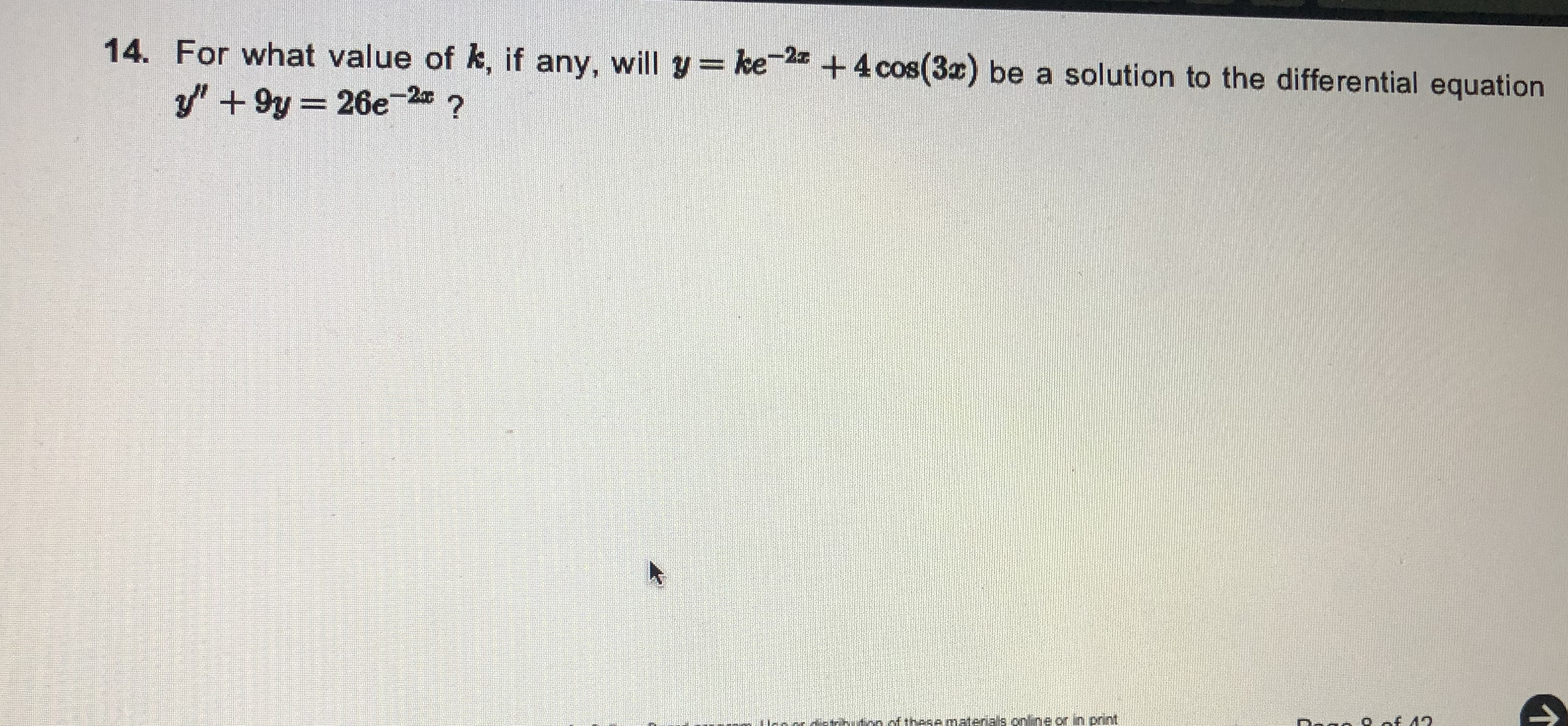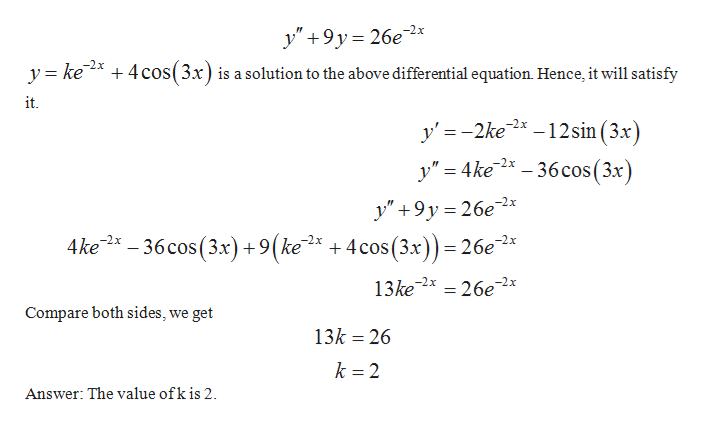# 14. For what value of k, if any, will y= ke+4 cos(3x) be a solution to the differential equationy" + 9y = 26e-2 ?of these materials online or in print

Question
155 views

Please look at the picture for the problem.help_outlineImage Transcriptionclose14. For what value of k, if any, will y= ke +4 cos(3x) be a solution to the differential equation y" + 9y = 26e-2 ? of these materials online or in print fullscreen
check_circle

Step 1

The solutions to the differential equation are those that satisfies the e...help_outlineImage Transcriptionclose-2x y" +9y= 26e -2x + 4 cos(3x) is a solution to the above differential equation. Hence, it will satisfy y = ke it. y' = -2ke* – 12sin (3x) y" = 4ke* – 36cos(3x) y" +9y = 26e2* 4ke* - 36 cos (3x) +9(ke* + 4cos(3.x))= 26e* -2x -2x 13ke 2x = 26e2x Compare both sides, we get 13k = 26 k = 2 Answer: The value of k is 2. fullscreen

### Want to see the full answer?

See Solution

#### Want to see this answer and more?

Solutions are written by subject experts who are available 24/7. Questions are typically answered within 1 hour.*

See Solution
*Response times may vary by subject and question.
Tagged in